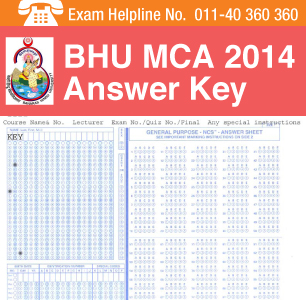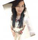BHU MCA 2014 Answer Key for three sets of the question papers is released in online mode by the exam conducting body Banaras Hindu University. The release of the official answer key is delayed by couple of days, as originally it was scheduled to be published on or before June 5, 2014.

Candidates will find the correct answers in the BHU MCA 2014 Answer Key and can tally their answer to the questions that were asked in the exam. Probable scores can also be calculated with the help of the Answer Key. The BHU MCA 2014 Answer Key can be downloaded from the same page.

For any doubts and queries students can call the BHU MCA Exam2014 Helpline at 011-40360360

Check here to download BHU MCA 2014 Answer Key for set 1

Check here to download BHU MCA 2014 Answer Key for set 2

Check here to download BHU MCA 2014 Answer Key for set 3

Candidates will be given five days (after the release of official answer key) to put forward complaint about the questions/answers. Candidates have to reach out the Office of the Controller of Examinations for filing complaint.

To file complaint, candidates have to mention the following details- their name, roll number, name of course, course code number, set number of question booklet (if any) and serial number of questions and its keys/correct answers.

Received complaints will be looked by Banaras Hindu University and its decision regarding Question(s)/ Key will be the final one.

Note: No request for evaluation of answer sheet or answer key will be accepted by BHU after the decision on complaint regarding BHU MCA 2014 Answer Key.

Stay connected to it.careers360.com for more news and feature articles on BHU MCA Entrance Exam 2014 Answer Key

BHU MCA 2014 Result

BHU MCA 2014 Cutoff

Questions related to BHU PET

Showing 218 out of 218 Questions
4 Views

i had PCM in 12th and completed BSc in botany and had chemistry in 1st and 2nd year of Bsc so am i eligilble for bhu pet exam for MSc in botany

Manidipa Kundu Student Expert 13th Oct, 2019hello,

for msc course in banaras hindu university eligibility criteria is students need to have a bachelors degree in relevant discipline. For M.Sc Zoology and M.Sc Botany, candidates must have also studied Chemistry at graduation level. as you have studied chemistry in b.sc course you are also eligible.

9 Views

Syllabus of bhu pet examination of physicsHello Debajyoti Maity

Score pattern of BHU PET

• BHU PET  questions are asked in MCQ Pattern.
• Each question will carry three marks.
• 120 questions are asked in the examination.
• Negative marking of one mark is there for each wrong answer.

Sections in the question paper are:

• Aptitude
• English Comprehension
• Language Proficiency
• Numerical and Quantitative Reasoning
• General Knowledge
• Current Affairs
• Analytical Abilities
• Mathematical Aptitude

Syllabus for English Comprehension and Language Proficiency

• Fill in the Blanks
• Improvement of Sentences
• Spotting the Error
• Synonyms &
• Spelling/ Detecting miss-spelt words
• One word Substitution
• Idioms & Phrases
• Close Passage & comprehension passage

Syllabus for Numerical and Quantitative Reasoning

• Decimals
• Exponents and Roots
• Fractions
• Integers
• Percent
• Ratio
• Real Numbers
• Coordinate Geometry
• Functions
• Graphs of Functions
• Operations with Algebraic Expressions
• Rules of Exponents
• Solving Linear Equations
• Solving Linear Inequalities
• Solving Quadratic Equations
• Circles
• Lines and Angles
• Polygons
• Three-Dimensional Figures
• Triangles
• Data Interpretation Examples
• Distributions of Data, Random Variables, and Probability Distributions
• Graphical Methods for Describing Data
• Numerical Methods for Describing Data
• Probability

Syllabus for General Knowledge and Current Affairs

• Latest Current Affairs
• Indian Constitution
• Indian History
• Indian Economy
• Indian Geography
• Indian Politics
• Indian Navy & Military

Syllabus of Mathematical Aptitude

• Fractions & Decimals,  Ratio & Proportion, Square Roots
• Simple & Compound Interest
• Time & Speed, Unitary Method,  Time & Work
• Profit & Loss
• Percentage &  Discount
• Simple Equations
• Polynomials
• Mean
• Median
• Mode
• Exponents
• Functions
• Sets
• Venn Diagrams
• Relations
• Functions
• Triangles
• Properties of Circles
• Probability
• Permutation and Combinations

Hope it helps you.

10 Views

Syllabus of bhu pet examination of physicsHello Debajyoti Maity

Score pattern of BHU PET

• BHU PET  questions are asked in MCQ Pattern.
• Each question will carry three marks.
• 120 questions are asked in the examination.
• Negative marking of one mark is there for each wrong answer.

Sections in the question paper are:

• Aptitude
• English Comprehension
• Language Proficiency
• Numerical and Quantitative Reasoning
• General Knowledge
• Current Affairs
• Analytical Abilities
• Mathematical Aptitude

Syllabus for English Comprehension and Language Proficiency

• Fill in the Blanks
• Improvement of Sentences
• Spotting the Error
• Synonyms &
• Spelling/ Detecting miss-spelt words
• One word Substitution
• Idioms & Phrases
• Close Passage & comprehension passage

Syllabus for Numerical and Quantitative Reasoning

• Decimals
• Exponents and Roots
• Fractions
• Integers
• Percent
• Ratio
• Real Numbers
• Coordinate Geometry
• Functions
• Graphs of Functions
• Operations with Algebraic Expressions
• Rules of Exponents
• Solving Linear Equations
• Solving Linear Inequalities
• Solving Quadratic Equations
• Circles
• Lines and Angles
• Polygons
• Three-Dimensional Figures
• Triangles
• Data Interpretation Examples
• Distributions of Data, Random Variables, and Probability Distributions
• Graphical Methods for Describing Data
• Numerical Methods for Describing Data
• Probability

Syllabus for General Knowledge and Current Affairs

• Latest Current Affairs
• Indian Constitution
• Indian History
• Indian Economy
• Indian Geography
• Indian Politics
• Indian Navy & Military

Syllabus of Mathematical Aptitude

• Fractions & Decimals,  Ratio & Proportion, Square Roots
• Simple & Compound Interest
• Time & Speed, Unitary Method,  Time & Work
• Profit & Loss
• Percentage &  Discount
• Simple Equations
• Polynomials
• Mean
• Median
• Mode
• Exponents
• Functions
• Sets
• Venn Diagrams
• Relations
• Functions
• Triangles
• Properties of Circles
• Probability
• Permutation and Combinations

Hope it helps you.

10 Views

Syllabus of bhu pet examination of physics

Ronak Gala Student Expert 9th Oct, 2019The following table shows the important topics of each and every subject that can be asked in the entrance test:

Section Topic
English Comprehension Fill in the Blanks
Improvement of Sentences
Spotting the Error
Synonyms & Antonyms
Spelling/ Detecting miss-spelt words
One word Substitution
Idioms & Phrases
Close Passage & comprehension passage
Quantitative Reasoning Decimals
Exponents and Roots
Fractions
Integers
Percent
Ratio
Real Numbers
Coordinate Geometry
Functions
Graphs of Functions
Operations with Algebraic Expressions
Rules of Exponents
Solving Linear Equations
Solving Linear Inequalities
Circles
Lines and Angles
Polygons
Three-Dimensional Figures
Triangles
Data Interpretation Examples
Distributions of Data, Random Variables, and Probability Distributions
Graphical Methods for Describing Data
Numerical Methods for Describing Data
Probability
General Knowledge Latest Current Affairs
Indian Constitution
Indian History
Indian Economy
Indian Geography
Indian Politics
Indian Navy & Military
Mathematical Aptitude Fractions & Decimals, Ratio & Proportion, Square Roots
Simple & Compound Interest
Time & Speed, Unitary Method, Time & Work
Profit & Loss
Percentage & Discount
Simple Equations
Polynomials
Exponents
Functions
Sets
Venn Diagrams
Relations
Functions
Triangles
Properties of Circles
Mean
Median
Mode
Probability
Permutation and Combinations
7 Views

Syllabus of bhu pet examination of physicsHello Debajyoti Maity

Score pattern of BHU PET

• BHU PET  questions are asked in MCQ Pattern.
• Each question will carry three marks.
• 120 questions are asked in the examination.
• Negative marking of one mark is there for each wrong answer.

Sections in the question paper are:

• Aptitude
• English Comprehension
• Language Proficiency
• Numerical and Quantitative Reasoning
• General Knowledge
• Current Affairs
• Analytical Abilities
• Mathematical Aptitude

Syllabus for English Comprehension and Language Proficiency

• Fill in the Blanks
• Improvement of Sentences
• Spotting the Error
• Synonyms &
• Spelling/ Detecting miss-spelt words
• One word Substitution
• Idioms & Phrases
• Close Passage & comprehension passage

Syllabus for Numerical and Quantitative Reasoning

• Decimals
• Exponents and Roots
• Fractions
• Integers
• Percent
• Ratio
• Real Numbers
• Coordinate Geometry
• Functions
• Graphs of Functions
• Operations with Algebraic Expressions
• Rules of Exponents
• Solving Linear Equations
• Solving Linear Inequalities
• Solving Quadratic Equations
• Circles
• Lines and Angles
• Polygons
• Three-Dimensional Figures
• Triangles
• Data Interpretation Examples
• Distributions of Data, Random Variables, and Probability Distributions
• Graphical Methods for Describing Data
• Numerical Methods for Describing Data
• Probability

Syllabus for General Knowledge and Current Affairs

• Latest Current Affairs
• Indian Constitution
• Indian History
• Indian Economy
• Indian Geography
• Indian Politics
• Indian Navy & Military

Syllabus of Mathematical Aptitude

• Fractions & Decimals,  Ratio & Proportion, Square Roots
• Simple & Compound Interest
• Time & Speed, Unitary Method,  Time & Work
• Profit & Loss
• Percentage &  Discount
• Simple Equations
• Polynomials
• Mean
• Median
• Mode
• Exponents
• Functions
• Sets
• Venn Diagrams
• Relations
• Functions
• Triangles
• Properties of Circles
• Probability
• Permutation and Combinations

Hope it helps you.

Top
The question have been saved in answer later, you can access it from your profile anytime. Access now